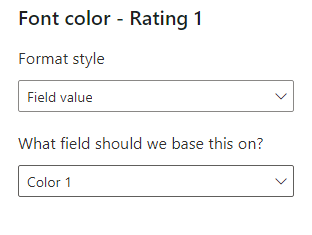cancel
Showing results for
Did you mean:Regular Visitor

## Looking up value from related table matching 2 columns

Evening,

I'm trying to add a value from a related table based on 2 columns values.

First Table (where I need the new column populated with coloured answer)

 Unique Company Rating 1 Rating 2 Rating 3 Overall A (rating) (rating) (rating) (rating) B (rating) (rating) (rating) (rating)

Related Table

 Unique Company Category Rating A Rating 1 Good A Rating 2 Satisfactory A Rating 3 Very Good A Overall Good B Rating 1 Satisfactory B Rating 2 Adequate B Rating 3 Good B Overall Satisfactory

I have tried using IF, Lookup and Switch but cannot get it quite right due to the multiple rows returning a result and breaking the query.

Any help appreciated

1 ACCEPTED SOLUTIONCommunity Support

Hi, @herbs

You can try the following methods.

Column:

``````Rating 1 =
CALCULATE(MAX('Table 1'[Rating]),FILTER('Table 1',[Unique Company]=EARLIER('Table 2'[Unique Company])&&[Category]="Rating 1"))``````
``````Rating 2 =
CALCULATE(MAX('Table 1'[Rating]),FILTER('Table 1',[Unique Company]=EARLIER('Table 2'[Unique Company])&&[Category]="Rating 2"))``````
``````Rating 3 =
CALCULATE(MAX('Table 1'[Rating]),FILTER('Table 1',[Unique Company]=EARLIER('Table 2'[Unique Company])&&[Category]="Rating 3"))``````
``````Overall =
CALCULATE(MAX('Table 1'[Rating]),FILTER('Table 1',[Unique Company]=EARLIER('Table 2'[Unique Company])&&[Category]="Overall"))``````Measure:

``````Color 1 =
IF(SELECTEDVALUE('Table 2'[Rating 1])="Good","Blue",IF(SELECTEDVALUE('Table 2'[Rating 1])="Satisfactory","Magenta"))``````
``````Color 2 =
``````Color 3 =
IF(SELECTEDVALUE('Table 2'[Rating 3])="Very Good","Green",IF(SELECTEDVALUE('Table 2'[Rating 3])="Good","Red"))``````
``````Color 4 =
IF(SELECTEDVALUE('Table 2'[Overall])="Good","Orange",IF(SELECTEDVALUE('Table 2'[Overall])="Satisfactory","Black"))``````Is this the result you expect?Best Regards,

Community Support Team _Charlotte

If this post helps, then please consider Accept it as the solution to help the other members find it more quickly.

5 REPLIES 5Regular Visitor

Thanks will give this a go and report backCommunity Support

Hi, @herbs

You can try the following methods.

Column:

``````Rating 1 =
CALCULATE(MAX('Table 1'[Rating]),FILTER('Table 1',[Unique Company]=EARLIER('Table 2'[Unique Company])&&[Category]="Rating 1"))``````
``````Rating 2 =
CALCULATE(MAX('Table 1'[Rating]),FILTER('Table 1',[Unique Company]=EARLIER('Table 2'[Unique Company])&&[Category]="Rating 2"))``````
``````Rating 3 =
CALCULATE(MAX('Table 1'[Rating]),FILTER('Table 1',[Unique Company]=EARLIER('Table 2'[Unique Company])&&[Category]="Rating 3"))``````
``````Overall =
CALCULATE(MAX('Table 1'[Rating]),FILTER('Table 1',[Unique Company]=EARLIER('Table 2'[Unique Company])&&[Category]="Overall"))``````Measure:

``````Color 1 =
IF(SELECTEDVALUE('Table 2'[Rating 1])="Good","Blue",IF(SELECTEDVALUE('Table 2'[Rating 1])="Satisfactory","Magenta"))``````
``````Color 2 =
``````Color 3 =
IF(SELECTEDVALUE('Table 2'[Rating 3])="Very Good","Green",IF(SELECTEDVALUE('Table 2'[Rating 3])="Good","Red"))``````
``````Color 4 =
IF(SELECTEDVALUE('Table 2'[Overall])="Good","Orange",IF(SELECTEDVALUE('Table 2'[Overall])="Satisfactory","Black"))``````Is this the result you expect?Best Regards,

Community Support Team _Charlotte

If this post helps, then please consider Accept it as the solution to help the other members find it more quickly.Regular Visitor

Hello

Unfortunately it doesn't work which i think is due to the table it is going into rather than the formula itself (creating a circular reference)

To help:

Table "Existing" as it looks currently

 Unique Company A B

Table "Ratings" as it looks currrently

 Unique Company Category Rating A Care Good A Team Outstanding A Happy Good B Care Adequate B Team Adequate B Happy Poor C Care Outstanding C Team Outstanding C Happy Good

I need table "Existing" to look like

 Uniqueness Company Care Team Happy A Good Outstanding Good B Adequate Adequate Poor C Outstanding Outstanding Good

Thanks again for your help so farSuper User

Hi,

I am not sure if I understood your question correctly, but please check the below picture and the attached pbix file.

It is for creating a new table.``````New Table =
VALUES ( Data[Unique Company] ),
"@Rating 1",
CALCULATE (
MAXX ( FILTER ( Data, Data[Category] = "Rating 1" ), Data[Rating] )
),
"@Rating 2",
CALCULATE (
MAXX ( FILTER ( Data, Data[Category] = "Rating 2" ), Data[Rating] )
),
"@Rating 3",
CALCULATE (
MAXX ( FILTER ( Data, Data[Category] = "Rating 3" ), Data[Rating] )
),
"@Overall", CALCULATE ( MAXX ( FILTER ( Data, Data[Category] = "Overall" ), Data[Rating] ) )
)``````

If this post helps, then please consider accepting it as the solution to help other members find it faster, and give a big thumbs up.Regular Visitor

Hi,

I have the 2 tables already - its the lookup query that is needed.

ThanksAnnouncements#### Exclusive opportunity for Women!

Join us for a free, hands-on Microsoft workshop led by women trainers for women where you will learn how to build a Dashboard in a Day!#### Power Platform Conference-Power BI and Fabric Sessions

Join us Oct 1 - 6 in Las Vegas for the Microsoft Power Platform Conference.Top Solution Authors
Top Kudoed Authors
Users online (3,785)